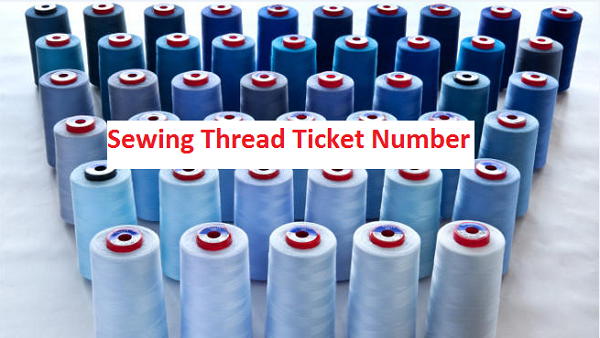# Ticket Number of Sewing Thread Used in Apparel Industry

By | January 28, 2016

### Ticket Number of Sewing Thread Used in Apparel Industry

Mayedul Islam
Merchandiser at Fashion Xpress Buying House.
Email: mayedul.islam66@gmail.com

#### Ticket Number:

Ticket number is a commercial numbering system. It is the manufacturer’s reference numbers for the size of a given sewing thread. Actually, the size or count of thread is called ticket number. It is denoted by tckt, Nm, Ne etc. Metric, cotton is the ticket numbering system followed in readymade garments sector.

You may follow Sewing Thread Consumption for Different Stitches#### Metric Ticket Number:

Metric count or number is the system of ticket numbering. It is denoted by Nm. This is the number of meters of thread per gram.

For example, one gram of single ply thread is 60m long. So that count or number of that thread is Nm 60/1. If the thread is double plied then metric ticket number would be Nm 60/2 and also the weight is 2gm for 60m long thread.

Double plied means two number of single yarn twisted together ply yarn. in the same way, metric ticket number (Nm) 90/3 means 3 single yarns are twisted together to form a thread, where each 90m length of single thread is 1gram and the weight of 90m length of 3 plied threads is 3 gram.

Metric ticket numbering has done by using the below formula:

Metric ticket number= Resultant count × 3

For easy understanding, the following table shows some example of metric ticket number.

 Metric number of thread (Nm) Metric ticket number of thread (Tkt. No.) 80/2 120 80/4 60 60/1 180 60/2 90

#### Cotton ticket number:

Cotton ticket number is used for cotton sewing thread. It is denoted by Ne.

For example, Ne 60/3 means sewing thread is 3 plied and the weight of each ply of 60 hank in 840yds is 1 pound.

Cotton ticket numbering has don by using the following equation:

Cotton ticket number= Resultant count × 3

To understand easily, the following table shows the example of cotton ticket number.

 Count of cotton thread (Ne) Cotton ticket number of thread (Tkt. No.) 40/2 60 40/3 40 40/4 30 60/2 90 60/3 60 60/4 45

1.Ikram Ullah
1.Mayedul Islam